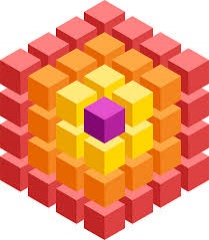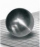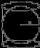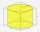# Inscribed sphere

How many percent of the cube volume takes the sphere inscribed into it?

p =  52.3599 %

### Step-by-step explanation:Did you find an error or inaccuracy? Feel free to write us. Thank you!Tips to related online calculators
Tip: Our volume units converter will help you with the conversion of volume units.

#### You need to know the following knowledge to solve this word math problem:

We encourage you to watch this tutorial video on this math problem:

## Related math problems and questions:

• Inscribed sphereHow many % of the volume of the cube whose edge is 6 meters long is a volume of a sphere inscribed in that cube?
• Cube into sphereThe cube has brushed a sphere as large as possible. Determine how much percent the waste was.
• Cube in ballCube is inscribed into the sphere of radius 181 dm. How many percent is the volume of cube of the volume of the sphere?
• Sphere fallHow many percent fall volume of a sphere if diameter decreases 3×?
• How many 9How many percent volume remain of a sphere if diameter decrease 3×?
• Sphere vs cubeHow many % of the surface of a sphere of radius 12 cm is the surface of a cube inscribed in this sphere?
• Equilateral cylinderA sphere is inserted into the rotating equilateral cylinder (touching the bases and the shell). Prove that the cylinder has both a volume and a surface half larger than an inscribed sphere.
• Cube in sphereThe cube is inscribed in a sphere with a radius r = 6 cm. What percentage is the volume of the cube from the volume of the ball?
• Reduce of the volumeCalculate how many % reduce the volume of the cube is we reduced length of each edge by 10%.
• Cube in a sphereThe cube is inscribed in a sphere with a volume 7253 cm3. Determine the length of the edges of a cube.
• Cube zoomHow many percents do we increase the volume and surface of the cube if we magnify its edge by 38 %?
• InscribedCube is inscribed in the cube. Determine its volume if the edge of the cube is 10 cm long.
• Cube in sphereThe sphere is an inscribed cube with an edge of 8 cm. Find the sphere's radius.
• Cube and waterHow many liters of water can fit into a cube with an edge length of 0.11 m?
• LatheCalculate the percentage of waste if the cube with 53 cm long edge is lathed to cylinder with a maximum volume.
• Sphere growthHow many times grow volume of sphere if diameter rises 10×?
• Cylinder in cubeInto a paper box in the shape of a cube with an edge of 10 cm is placed a can in the shape of a cylinder with a height of 10 cm and touching all the walls of the cube. What % of the volume of the cube can take up?